Home / Digital Circuits / One’s and Two’s Complement of Binary Number

# One’s and Two’s Complement of Binary Number

Want create site? Find Free WordPress Themes and plugins.

For the analysis of practical applications, it is necessary to do mathematical operations on binary numbers. This is the basis of all that a microprocessor does. In this section, we study how two binary numbers are added, how one is subtracted from the other. We also discuss how the negative of a binary number is formed.

Addition

The addition of two binary numbers is performed in the same way as we add two decimal numbers with the exception that the only nonzero element in the set of basic elements of the binary number system is the number 1. Thus, in the same way, that in decimal numbers if we add 1 to 9, the answer is 10, in binary numbers if we add 1 to 1, the answer is 10. That is, the addition of 1 and 1 is a 0 with a 1 carried over to the next digit on the left. Also, practically, which is the way this operation is performed in microprocessors, only two numbers are added together at a time. We consider the following three examples. Assume unsigned numbers.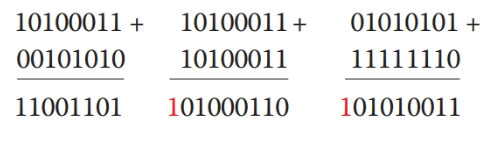Because any binary numbers are practically the contents of a register, only a limited number of bits are available. This is because of the hardware, which determines the bit size to be 8, 10, 12, or some other.

In the result of adding two numbers, you see that the very last digit on the left is shown in red when it happens to exist. This digit (bit) is ignored. There is physically no place for this bit to go to. It is either not significant (in a subtraction operation, as you will see next), or it is an indication of overflow (as shown above).

Overflow is a situation where a number is larger than the capacity of the machine. When there is an overflow situation, the last bit is ignored, but it signals out a flag.

A flag is an indicator of an undesirable or fault situation in digital systems. A message will be issued when a flag is signaled. An example is when you have a division by zero in your calculator.

Overflow: State of reaching a value beyond the capacity of a digital device in terms of the number of bits.

Flag: Representation of the occurrence of some unusual or unwanted situation in digital circuits and computer terminology.

Subtraction

To subtract a number B from number A the common method is to add A to the negative of B.

$A-B=A+\left( -B \right)$

The subtraction, then, changes to an addition operation. Then, in the same way, as discussed above the two numbers are added.

Consider for example subtraction of 44 (0010, 1100b) from 108 (0110, 1100b) in binary operation. Instead, we add −44 (1101, 0100b) to 108You can easily check that 108 − 44 = 64, and the resultant binary number (0100, 0000b) is 64. Also, you can verify the number for −44 to be correct. If it is added to 44, the resultant is 0.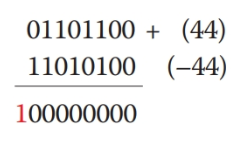Note that in both cases the very last digit, shown in red, does not play any role and is ignored.

## One’s Complement of a Binary Number

The one’s complement of a binary number is used to find the two’s complement of a number, which is used to find the negative of that number. Note that negatives are valid with signed numbers.

The one’s complement of a number is obtained by switching each bit to its opposite value, 1’s to 0’s and 0’s to 1’s. For example, the one’s complement of 0110, 1010 (106) is 1001, 0101.

One’s complement: The result of swapping each bit value to its complement in a binary number.

Two’s complement: A binary number obtained by adding 1 to the one’s complement of a binary number.

## Two’s Complement of a Binary Number

Two’s complement of a binary number is obtained by adding 1 to the one’s complement of that number. For instance,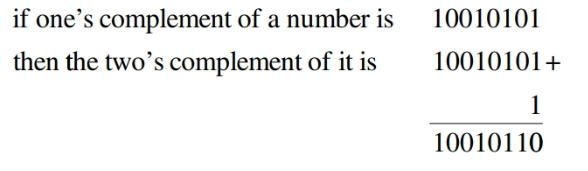The two’s complement defines the negative of a number. Therefore, the negative of 0110, 1010b is 1001, 0110b. We can check this by adding the two numbers together.

$\begin{matrix}01101010+ \\10010110 \\—— \\100000000 \\\end{matrix}$

Example 1

Find the negative of the binary number 1110, 0111.

Solution

First, note that this number is already negative because its most significant bit is 1. The negative of a negative number is positive. No matter if the number is initially positive or negative, the two steps to be taken are

Step 1. Find the one’s complement of the number One’s complement of 1110, 0111 is 0001, 1000.

Step 2. Find the two’s complement of the number (or, add 1 to the one’s complement)

$\text{0001,100}{{\text{0}}_{\text{b}}}\text{ + 1 = 0001,100}{{\text{1}}_{\text{b}}}$

The answer, thus, is 0001, 1001. Verifying the result:

$\begin{matrix}11100111+ \\00011001 \\—— \\100000000 \\\end{matrix}$

The given numbers was −25. You cannot say that easily just from the number directly by looking at the digits, except that one has a look-up table showing all the numbers in the range.

Figure 1 shows some numbers between −128 and −1. You may notice a pattern to determine the numbers for 8 bits as shown in the table. On the basis of this pattern, find the decimal magnitude of the binary number leaving out the leftmost bit, and subtract 128 from that number. For other bit numbers, the value to subtract (128 in this case) must be changed accordingly.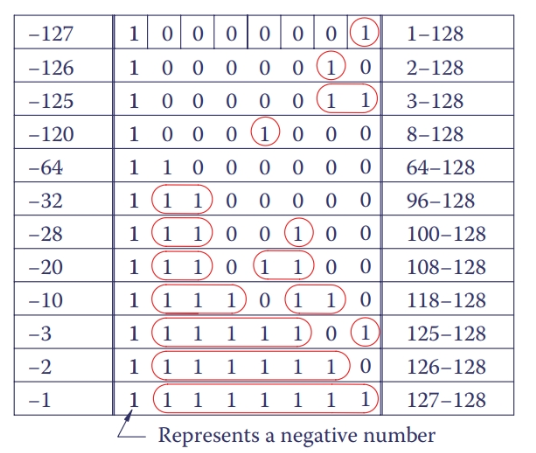Figure 1 Negative binary numbers.

The two’s complement of a binary number defines the negative of that number.

## Multiplication and Division of Unsigned Binary Numbers

Multiplication of binary numbers is very easy. This is the way multiplication is performed in a microprocessor. First, consider multiplication by 10b and 100b, which is, in fact, multiplication by 2 and 4. Consider 0011, 1011b (always assume a limited number of bits, here 8). Assume an unsigned number.

\begin{align}& \begin{matrix}0011,{{1011}_{b}}\times {{10}_{b}}=0111,{{0110}_{b}} & {} & \left( 59\times 2=118 \right) \\\end{matrix} \\& \begin{matrix}0011,{{1011}_{b}}\times {{100}_{b}}=1110,{{1100}_{b}} & {} & \left( 59\times 4=236 \right) \\\end{matrix} \\\end{align}

As in the case of decimal numbers 1 or 2, 0’s are added to the right side of the number for multiplication by 10b and 100b, respectively. Because the number of bits must remain the same, this operation is like bringing the 0’s from the left hand to the right hand. Figure 2 depicts this. This operation of moving 0’s to the right is called rotation.

Rotation: (in binary number operations) Operation of moving one or more leftmost zeros in a binary number with a limited number of digits to the far right. This implies multiplying that number by 10b, 100b, etc.

Rotation to the right is performed for multiplication, 1 bit for multiplication by 10b, 2 bits for multiplication by 100b, and so on. Note that here if multiplication is continued, then eventually overflow happens when the result becomes larger than a machine can handle (here because there are only 8 bits, not many numbers can be handled, but in a machine, with 128 bits the operation can go on much further).

For multiplication by numbers like 3 (11b) and 6 (110b) and so forth, these numbers are broken into their components. For example,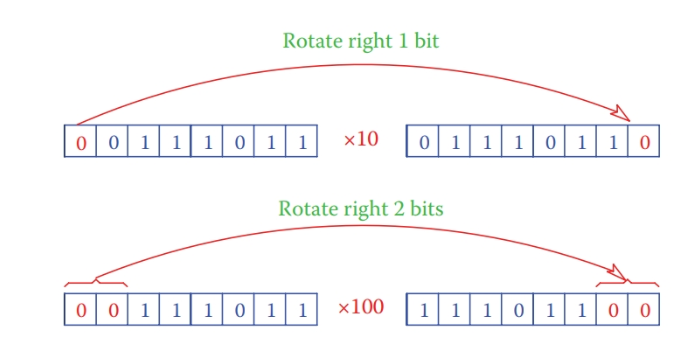Figure 2 Multiplication of a number by 10b and 100b.

${{11}_{b}}={{10}_{b}}+1$

Thus, multiplication of a binary number by 11b includes multiplication of that number by 10b, as above, and multiplication of the number by 1(multiplication by 1 is trivial and does not change the number), and adding the results. Similarly, multiplication by 110b includes multiplication by 100b plus multiplication by 10b,

$\text{11}{{\text{0}}_{\text{b}}}\text{ = 10}{{\text{0}}_{\text{b}}}\text{ + 1}{{\text{0}}_{\text{b}}}$

both of which we have already seen. The results of the two multiplications then must be added together. Multiplication by all other numbers can be treated the same way.

Example 2

Multiply the 10-bit binary number 00, 0110, 0111b by 7.

Solution

Multiplication of the number by 7 consists of multiplication by 4 + 2 + 1. Because 7 = 111b, then the number must be multi- plied by 100b, multiplied by 10b, and multiplied by 1b followed by adding all the results.

\begin{align}& \text{00,0110,011}{{\text{1}}_{\text{b}}}\times {{100}_{b}}=\text{01,1001,110}{{\text{0}}_{b}} \\& \text{00,0110,011}{{\text{1}}_{\text{b}}}\times {{10}_{b}}=\text{00,1100,111}{{\text{0}}_{b}} \\& \text{00,0110,011}{{\text{1}}_{\text{b}}}\times {{1}_{b}}=\text{00,0110,011}{{\text{1}}_{b}} \\\end{align}

The final result is

$\text{01,1001,110}{{\text{0}}_{\text{b}}}\text{+ 00,1100,111}{{\text{0}}_{\text{b}}}\text{+ 00,0110,011}{{\text{1}}_{\text{b}}}\text{ =10,1101,000}{{\text{1}}_{\text{b}}}$

You may want to check the results with decimal numbers. The initial number was 103, and the final answer is 721, which is 103 × 7.

In conjunction with multiplication is division. For division, numbers are shifted right. Shift right is shown in Figure 3. In dividing integer numbers, round-off errors can occur, as can be seen in the figure.

Shift right: (in binary number operations) Operation of moving all digits of a binary number (with a limited number of digits) to the right by one or more places. The left- most places are then filled with zeros. This implies dividing that number by 10b, 100b, etc. The rightmost digits are moved out and introduce the round-off error that occurs in the division.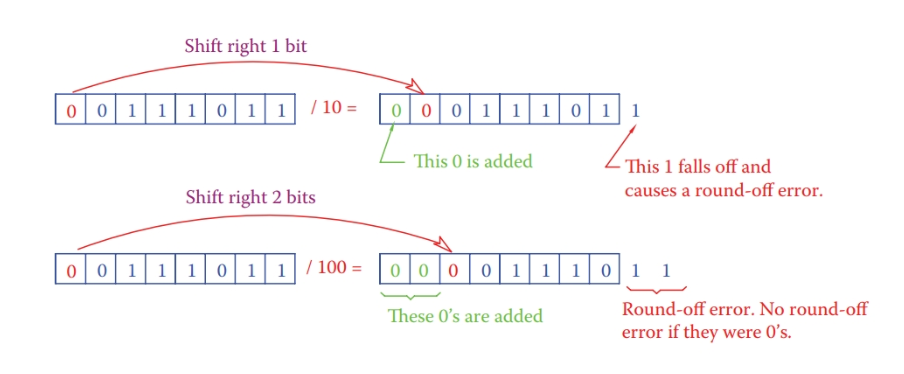Figure 3 Division in binary unsigned numbers.

Did you find apk for android? You can find new Free Android Games and apps.

### About Ahmad FaizanMr. Ahmed Faizan Sheikh, M.Sc. (USA), Research Fellow (USA), a member of IEEE & CIGRE, is a Fulbright Alumnus and earned his Master’s Degree in Electrical and Power Engineering from Kansas State University, USA.